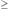Students / SubjectsRevealed Preference (WARP, SARP, and GARP) Revealed preference theory provides a direct test of the utility model of preferences. A very simple application of the concepts of revealed preference was used to demonstrate that the two choices made in the example of inconsistent choices could not be produced by a consumer who chooses the consumption levels to maximize a utility function. That example though is the simplest case of choices that are inconsistent with a utility function. The property that is violated in that example is called the Weak Axiom of Revealed Preference (WARP). Before providing the definition of WARP, some background and notation is helpful. We say that a utility function u(x) rationalizes the choice x if at the given prices, the chosen level of consumption has utility that is at least as high as any other available choice. Stated formally, if prices are given by the vector pi = (p1i, p2i, ..., pni), and if the chosen consumption levels of goods 1, 2, ..., n are xi = (x1i, x2i, ..., xni), then u(xi)u(x) for any consumption level x such that pi xipi x. When xi is chosen and pi xipi x so that x is an available choice, we say that xi is directly revealed preferred to x. This is written xi R0 x. Weak Axiom of Revealed Preference (WARP) WARP If xi R0 xj and xi is not equal to xj, then it is not the case that xj R0 xi. In the example of inconsistent choices, we had both xa R0 xb and xb R0 xa. The analysis of the inconsistency in that example shows directly why these two choices are inconsistent with the existence of a utility function. Strong Axiom of Revealed Preference (SARP) WARP is one implication of choices that are consistent with a utility function, but there are other implications as well. Another implaication, called the Strong Axiom of Revealed Preference (SARP) is closely related to WARP. If x 1 R0 x 2, x 2 R0 x 3, and so on until x n - 1 R0 x n, then we say that x 1 is revealed preferred to x n. This is written as x 1 R x n. The primary difference between SARP and WARP is that SARP not only rules out two choices that are both directly revealed preferred to one another, but it also rules out chains of choices that ultimately lead to two choices that are each revealed preferred to one another. SARP If xi R xj and xi is not equal to xj, then it is not possible to have xj R xi. In the example below, there are three different prices, and three choices. For each pair of prices and choices, there is no violation of WARP, but there is a cycle that violates SARP. Example: The first price and associated consumption choices are p1 = (2, 3, 3) and x1 = (3, 1, 7). The second price and choices are p2 = (3, 2, 3) and x2 = (7, 3, 1), and the third pair are p3 = (3, 3, 2) and x3 = (1, 7, 3). None of these price and consumption pairs violate WARP. This can be verified directly by observing that for the price p1, x2 is available but x3 is not available. Since x1 is not available at the price p2, there is no violation of WARP that involves x1. When the price is p2, the consumption level x3 is affordable, but when the price is p3, x2 is not available, so there is no violation of WARP that involves x2. The problem with this set of choices is with the cycle x1 R0 x2, x2 R0 x3, and x3 R0 x1. The combination of the first two of these show that x1 R x3, and from the third, x3 R x1, so SARP is violated but WARP is not. This example leads to a problem similar to the example of inconsistent choices, and the demonstration that this example is inconsistent with the existence of a utility function that represents choices is similar. Generalized Axiom of Revealed Preference (GARP) SARP is very close to a characterization of the implications of utility maximization. If there is always a single consumption level that maximizes utility, then SARP does characterize all of the implications of utility maximization. The Generalized Axiom of Revealed Preference (GARP) covers the case when, for some price level, there may be more than one level of consumption that maximizes utility. In order to state GARP, one more term is useful. If pi xi > pi x and xi is the chosen consumption level, then xi is strictly directly revealed preferred to x. GARP If xi is revealed preferred to xj, then xi cannot be strictly directly revealed preferred to xi. The GARP Exercise The objective of the GARP exercise is to provide students with a price for each of several commodities, and a budget. The student is then asked to select a level of consumption for each of the commodities. This choice problem is repeated several times with different prices. The resulting choices can then be analyzed to determine whether the choices are consistent with the existence of a utility function, that is, to determine whether there is any violation of GARP. If there is a violation of GARP, the analysis reports the number of violations.